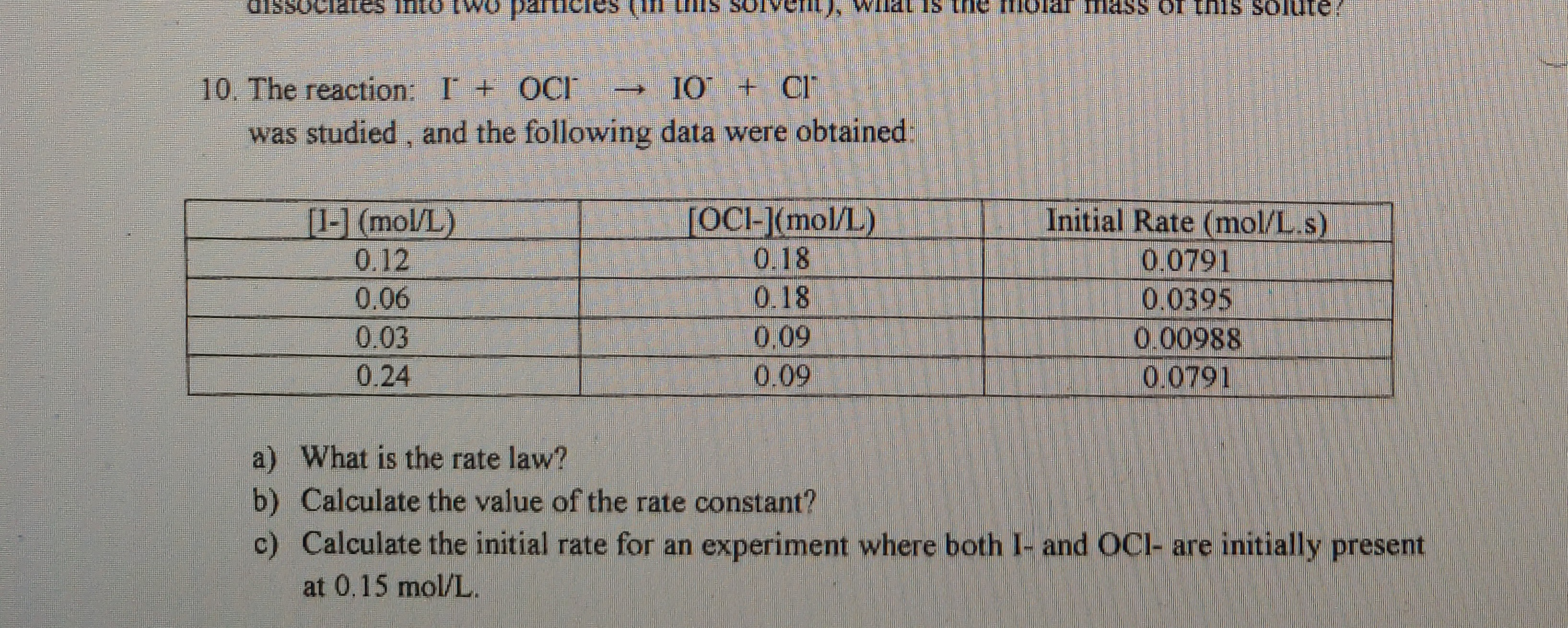# The reaction: I- + OCl- -- > IO- + Cl- and the following data were obtained: a) What is the rate law? b) Calculate the value of the rate constant c) Calculate the initial rate for an experiment where both I- and OCl- are initially present at 0.15 mol/L